### 3.2.1 Theory

#### 3.2.1.1 The harmonic series

The additive series of frequencies (i.e., the series that results from simply adding the same Hertz value repeatedly), which results in a string of intervals of decreasing size, is called the harmonic series: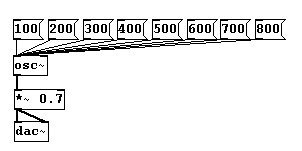You can also derive the series by repeating an experiment devised by Pythagoras (ca. 570-510 BCE) in which a string is divided into various proportions: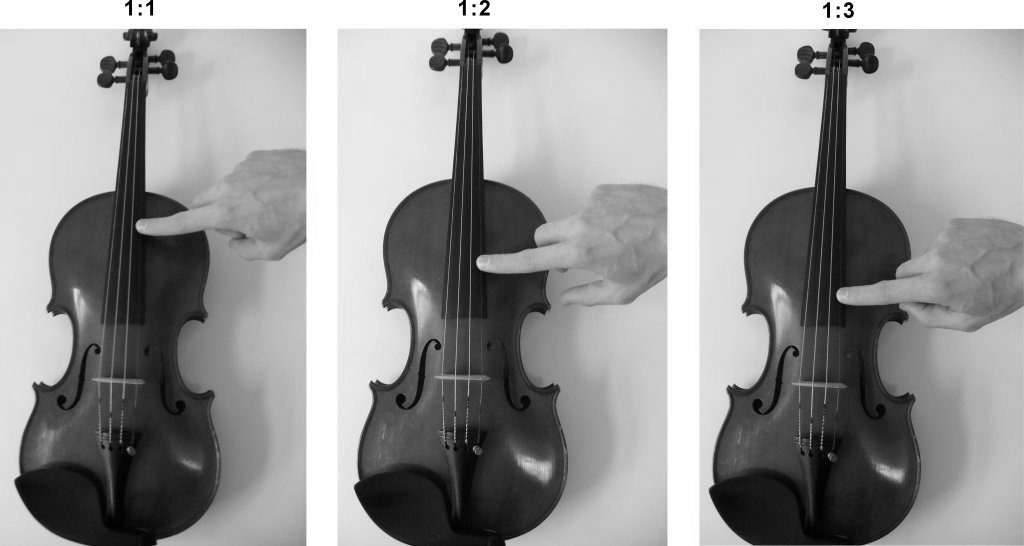The ratios describe the length of the two parts of the string in relation to each other.

When a string is bowed, it doesn't just vibrate as a whole, but also in every whole number proportion: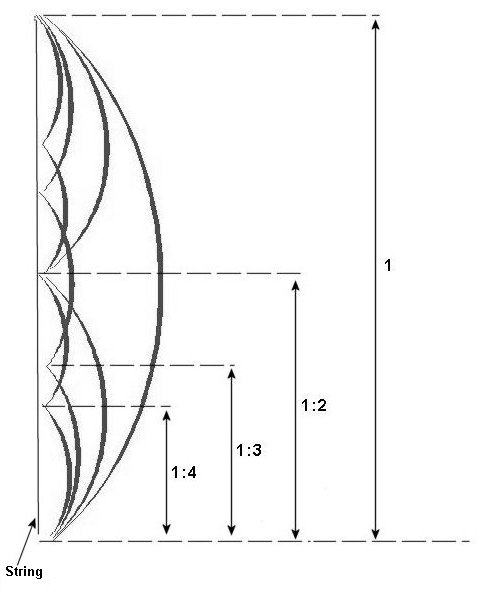Here the ratios describe the length of the vibrating section in relation to the length of the entire string.

All of these partial vibrations (called 'partials' or 'harmonics') result in sound as well, so every sound made on a string is in fact already a chord!

The special thing about this chord is that all of its pitches melt together, at least when their relative volumes decrease as the pitches get higher. Every natural sound has overtones. Due to characteristics inherent to the human ear, we hear all of these pitches as just one tone.

In contrast, the upper partials themselves (i.e., the partials above the fundamental) do not have any overtones. An isolated sound without overtones does not exist in nature, but such a thing can be created using electronic means. These are called sine tones, a name that stems from the shape of their waveform:Physicist Jean Baptiste Joseph Fourier (1768-1830) discovered that every periodic sound can be represented using only sine tones (of different frequency, amplitude, and phase), the sum of which is then identical with the original. Such an analysis and the corresponding mathematical process is called a Fourier analysis and Fourier transformation.

Using this principle, it is possible to create every periodic sound by layering many sine tones, a process called "additive synthesis".

In Pd, as already mentioned, "osc~" can be used to generate a sine tone. Sine tones are a very characteristic sound of electronic music, as they are produced and can only be produced using electronic means.

Using a number of "osc~" objects, whose frequencies form an additive series, you can create a chord based on the overtone series: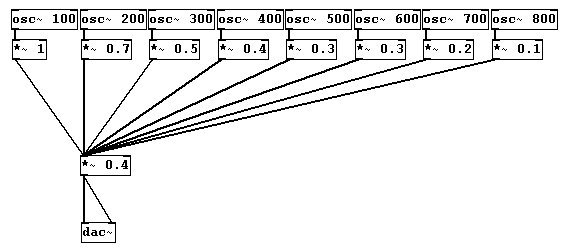Typically, amplitudes become smaller as the frequencies get larger in order for the chord to blend better (though for some instruments, it is characteristic for certain partials to be louder than those on either side of them, e.g., the clarinet). The arrangement and relative volumes of overtones determine a sound's color. You can also speak of its spectrum.

The fact that our ears blend the overtones together becomes clear when you change the fundamental frequency: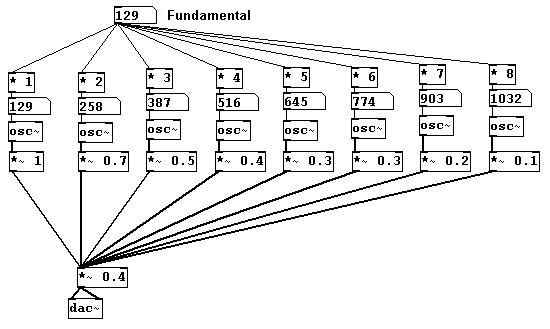We'll just use the first eight partials here. (N.B. The term 'partial' includes the fundamental whereas the term 'overtone' does not. In other words, the 1st partial = the fundamental frequency, 2nd partial = 1st overtone, 3rd partial = 2nd overtone, etc.)

Even if you leave out the lower partials, you hear the fundamental frequency as the fundamental when you change it:Our brain calculates the fundamental based on the remaining spectrum. This non-existent tone is called a residual tone.

### 3.2.2 Applications

#### 3.2.2.1 A random klangfarbe (German: sound color)

For the sake of space, this example has been limited to just the first seven partials: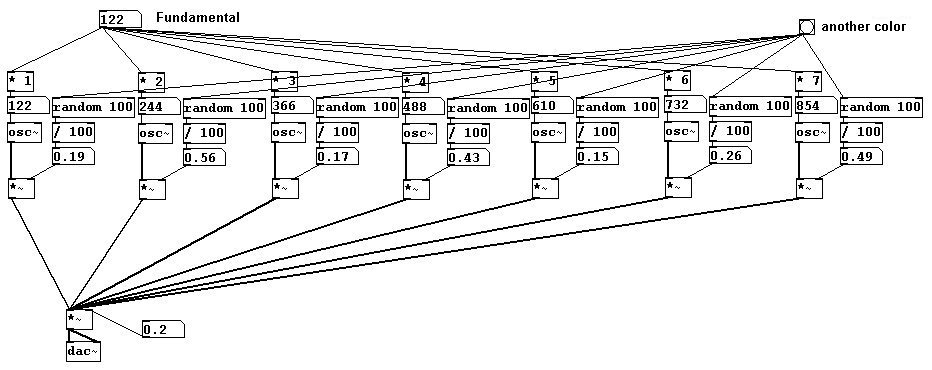#### 3.2.2.2 Changing one klangfarbe into another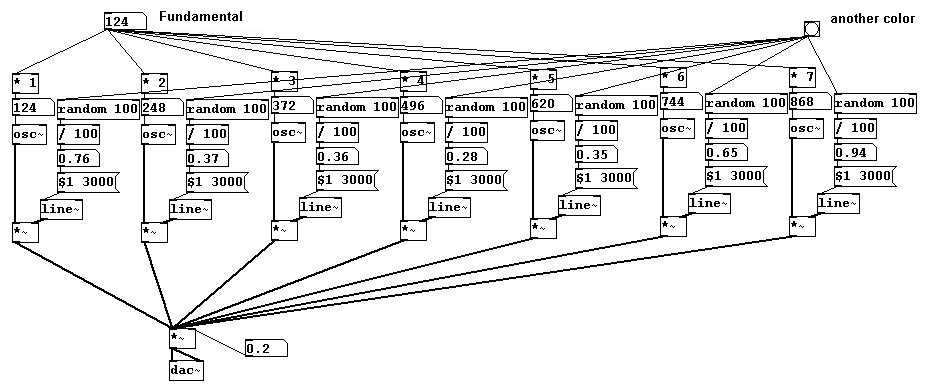#### 3.2.2.3 Natural vs. equal-tempered

Let's look at the difference between natural and equal-tempered intervals (first enter the fundamental frequency!):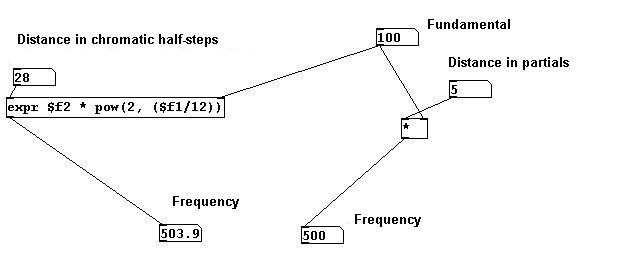Showing the difference between natural and equal-tempered tuning in cents (hundredths of a half-step):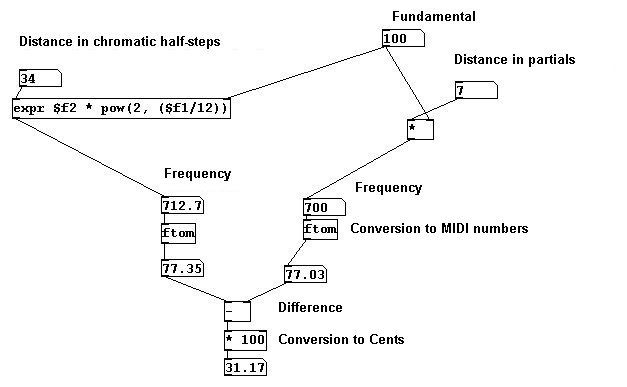You can see here: the 7th partial is 31 cents flatter than the equal-tempered seventh.

#### 3.2.2.4 More exercises

Create an overtone chord with manipulated overtones, i.e., with imprecise overtones.

### 3.2.3 Appendix

#### 3.2.3.1 Pd's limitations

The previous example of random klangfarbe reveals one of Pd's limitations: you can't randomly determine the number of oscillators. You have to at least determine the maximum first.

### 3.2.4 For those especially interested

#### 3.2.4.1 Studie II

One of the pioneering pieces in the history of electronic music is 'Studie II' by Karlheinz Stockhausen, written in 1954. This work uses only sine tones and mixtures thereof in non-tempered intervals. The author strongly recommends you analyze this piece!

#### 3.2.4.2 Composing with spectra

In the fourth chapter of his book "Audible Design", composer and theorist Trevor Wishart describes many possibilities for composing with spectra.